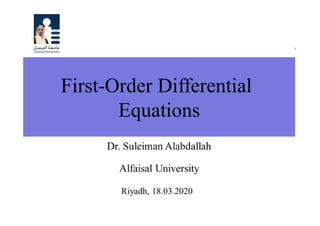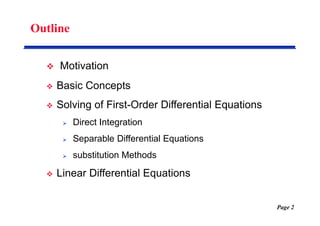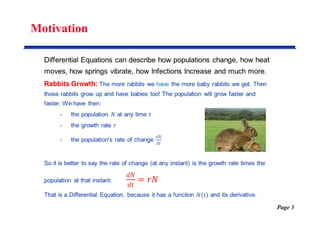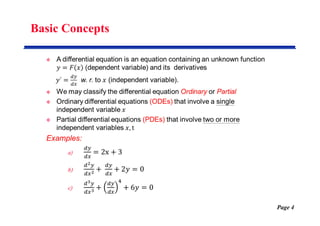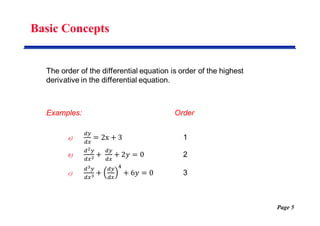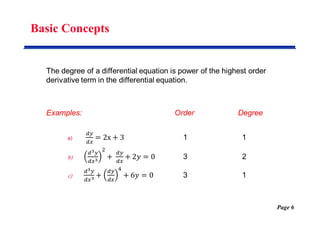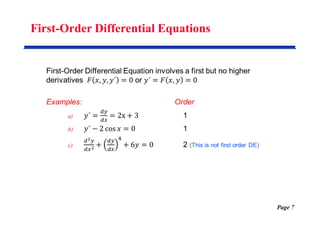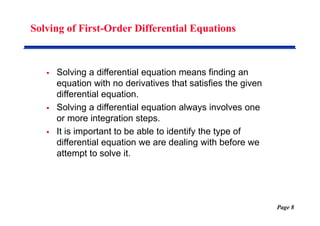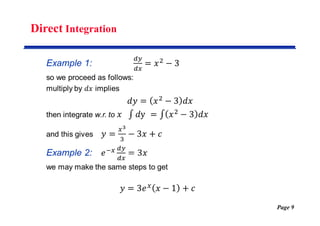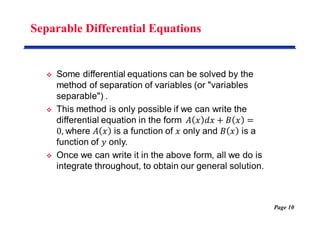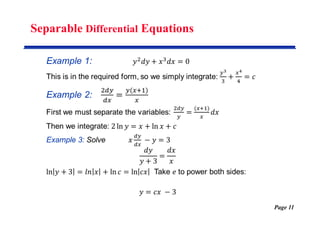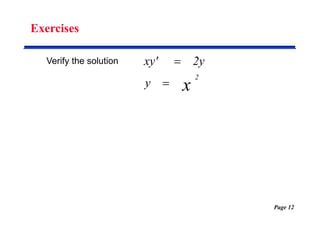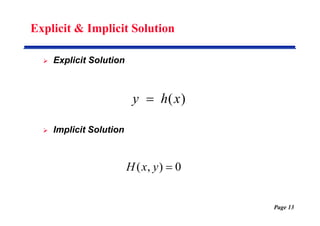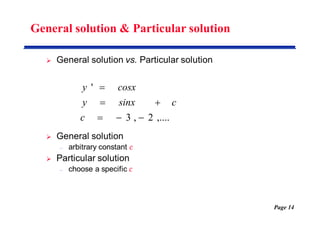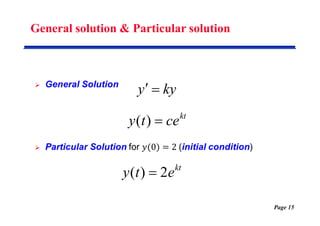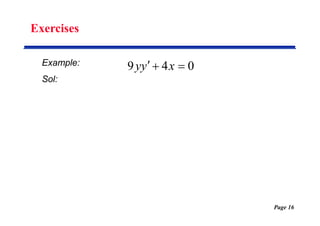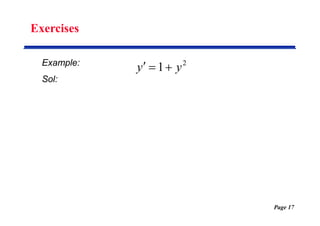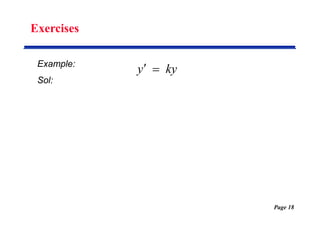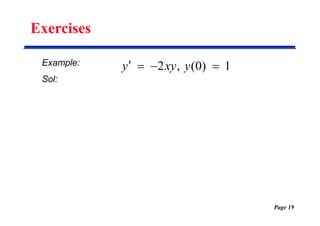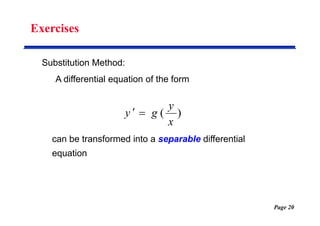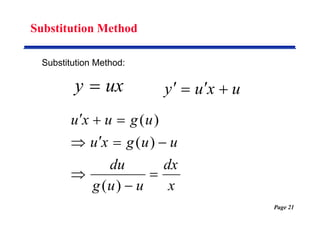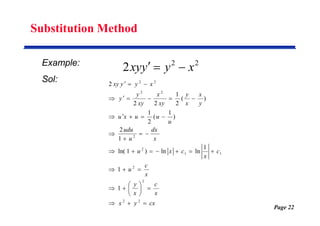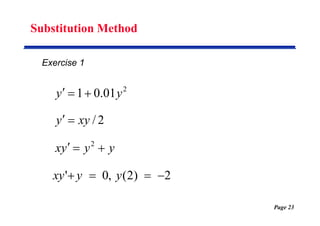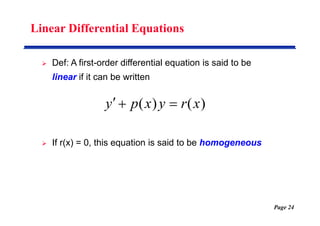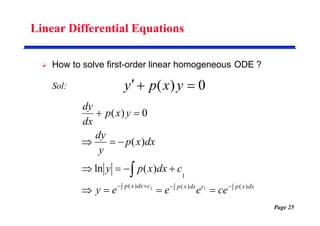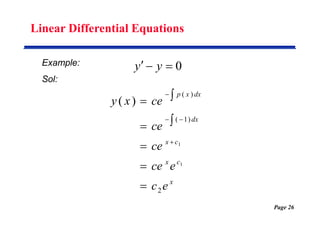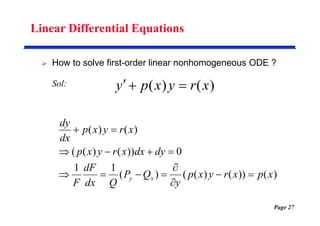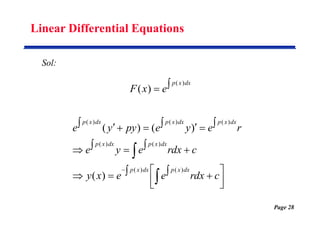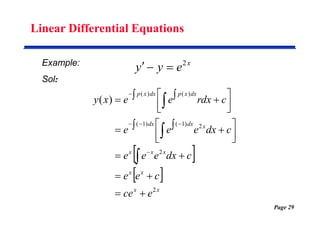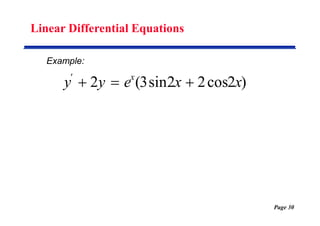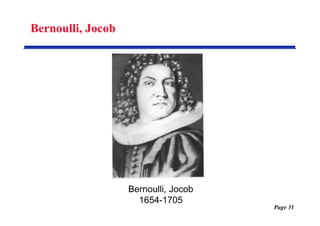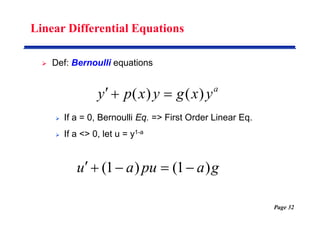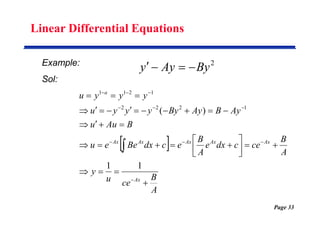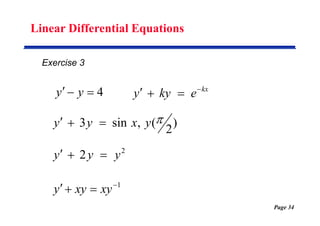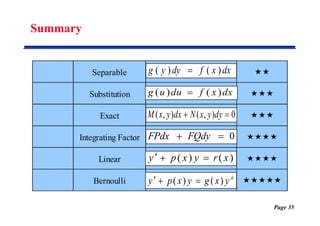1 of 35

### First Order Differential Equations

1. Page 1
2. Page 2 Outline  Motivation  Basic Concepts  Solving of First-Order Differential Equations  Direct Integration  Separable Differential Equations  substitution Methods  Linear Differential Equations
3. Page 3 Motivation
4. Basic Concepts Page 4
5. Basic Concepts Page 5
6. Basic Concepts Page 6
7. First-Order Differential Equations Page 7
8. Solving of First-Order Differential Equations  Solving a differential equation means finding an equation with no derivatives that satisfies the given differential equation.  Solving a differential equation always involves one or more integration steps.  It is important to be able to identify the type of differential equation we are dealing with before we attempt to solve it. Page 8
9. Direct Integration Page 9
10. Separable Differential Equations Page 10
11. Separable Differential Equations Page 11
12. Page 12 Exercises Verify the solution x 2 y 2y xy'  
13. Page 13 Explicit & Implicit Solution  Explicit Solution  Implicit Solution ) (x h y  0 ) , (  y x H
14. Page 14 General solution & Particular solution ,.... 2 , 3 '       c c sinx y cosx y
15. Page 15 General solution & Particular solution kt ce t y  ) ( kt e t y 2 ) (  ky y  
16. Page 16 Exercises Example: Sol: 0 4 9    x y y
17. Page 17 Exercises Example: Sol: 2 1 y y   
18. Page 18 Exercises Example: Sol: ky y  
19. Page 19 Exercises Example: Sol: 1 ) 0 ( , 2     y xy y
20. Page 20 Exercises Substitution Method: A differential equation of the form can be transformed into a separable differential equation ) ( x y g y  
21. Page 21 Substitution Method Substitution Method: ux y  u x u y     x dx u u g du u u g x u u g u x u           ) ( ) ( ) (
22. Page 22 Substitution Method Example: Sol: 2 2 2 x y y xy    cx y x x c x y x c u c x c x u x dx u udu u u u x u y x x y xy x xy y y x y y xy                                         2 2 2 2 1 1 2 2 2 2 2 2 1 1 1 ln ln ) 1 ln( 1 2 ) 1 ( 2 1 ) ( 2 1 2 2 2
23. Page 23 Substitution Method Exercise 1 2 01 . 0 1 y y    2 / xy y   y y y x    2 2 ) 2 ( , 0 '     y y xy
24. Page 24 Linear Differential Equations  Def: A first-order differential equation is said to be linear if it can be written  If r(x) = 0, this equation is said to be homogeneous ) ( ) ( x r y x p y   
25. Page 25 Linear Differential Equations  How to solve first-order linear homogeneous ODE ? Sol: 0 ) (    y x p y                      dx x p c dx x p c dx x p ce e e e y c dx x p y dx x p y dy y x p dx dy ) ( ) ( ) ( 1 1 1 ) ( ln ) ( 0 ) (
26. Page 26 Linear Differential Equations Example: Sol: 0    y y x c x c x dx dx x p e c e ce ce ce ce x y 2 ) 1 ( ) ( 1 1 ) (           
27. Page 27 Linear Differential Equations  How to solve first-order linear nonhomogeneous ODE ? Sol: ) ( ) ( x r y x p y    ) ( )) ( ) ( ( ) ( 1 1 0 )) ( ) ( ( ) ( ) ( x p x r y x p y Q P Q dx dF F dy dx x r y x p x r y x p dx dy x y              
28. Page 28 Linear Differential Equations Sol:   dx x p e x F ) ( ) (                            c dx r e e x y c dx r e y e r e y e py y e dx x p dx x p dx x p dx x p dx x p dx x p dx x p ) ( ) ( ) ( ) ( ) ( ) ( ) ( ) ( ) ( ) (
29. Page 29 Linear Differential Equations Example: Sol: x e y y 2        x x x x x x x x dx dx dx x p dx x p e ce c e e c dx e e e c dx e e e c dx r e e x y 2 2 2 ) 1 ( ) 1 ( ) ( ) ( ) (                                  
30. Page 30 Linear Differential Equations Example: ) 2 cos 2 2 sin 3 ( 2 x x e y y x '   
31. Page 31 Bernoulli, Jocob Bernoulli, Jocob 1654-1705
32. Page 32 Linear Differential Equations  Def: Bernoulli equations  If a = 0, Bernoulli Eq. => First Order Linear Eq.  If a <> 0, let u = y1-a a y x g y x p y ) ( ) (    g a pu a u ) 1 ( ) 1 (     
33. Page 33 Linear Differential Equations Example: Sol: 2 By Ay y       A B ce u y A B ce c dx e A B e c dx Be e u B Au u Ay B Ay By y y y u y y y u Ax Ax Ax Ax Ax Ax a                                               1 1 ) ( 1 2 2 2 1 2 1 1
34. Page 34 Linear Differential Equations Exercise 3 4    y y kx e ky y     2 2 y y y    1     xy xy y ) 2 ( , sin 3  y x y y   
35. Page 35 Summary Separable  Substitution  Exact  Integrating Factor  Linear  Bernoulli  dx x f dy y g ) ( ) (  dx x f du u g ) ( ) (  0 ) , ( ) , (   dy y x N dx y x M 0   FQdy FPdx ) ( ) ( x r y x p y    a y x g y x p y ) ( ) (   